# ID3 ALGORITHM

4 Jan 2016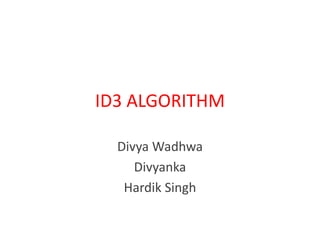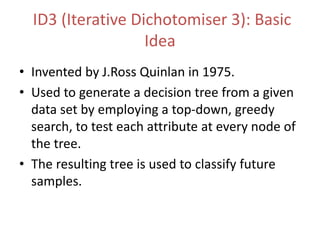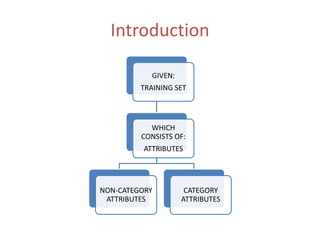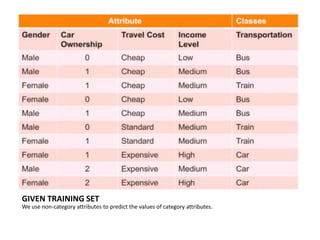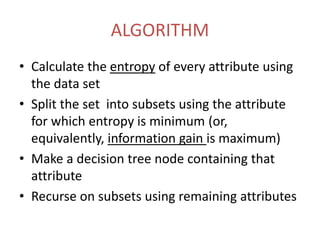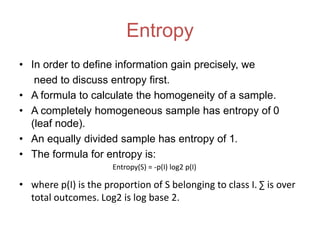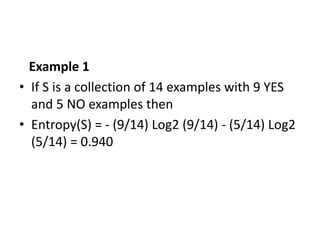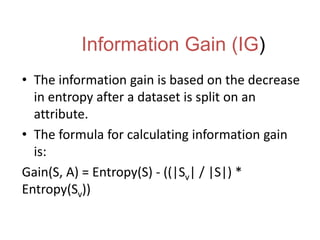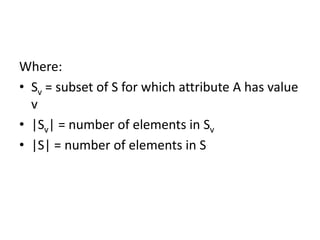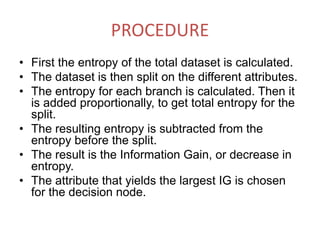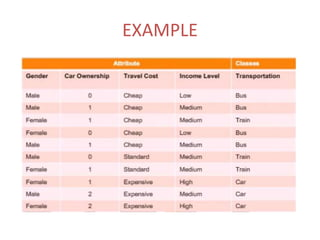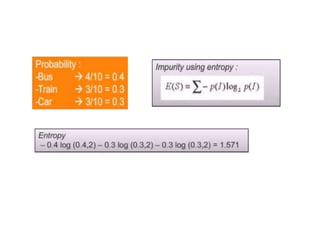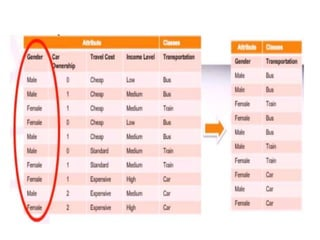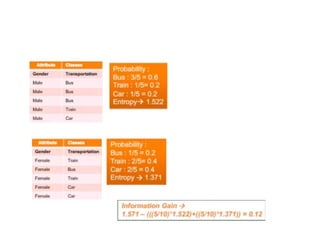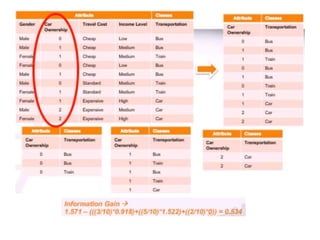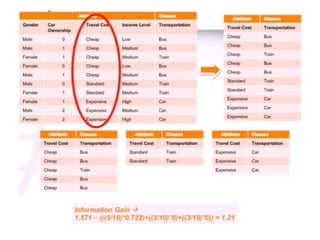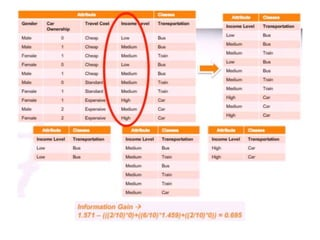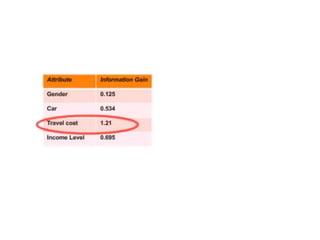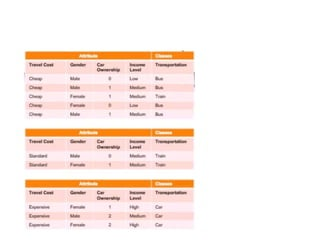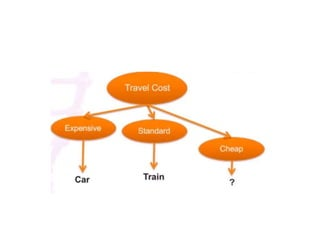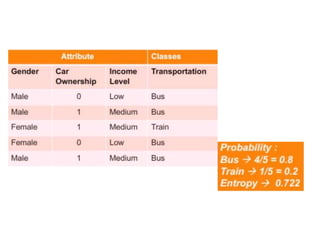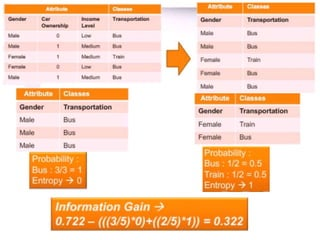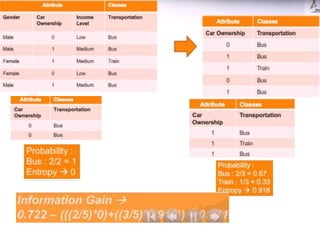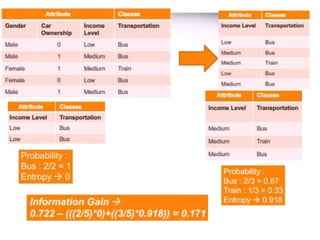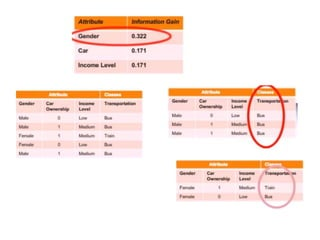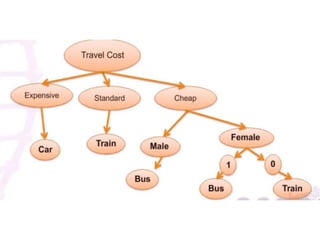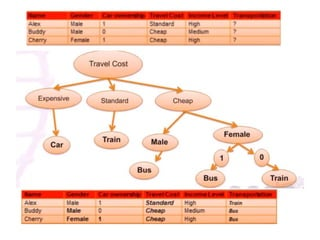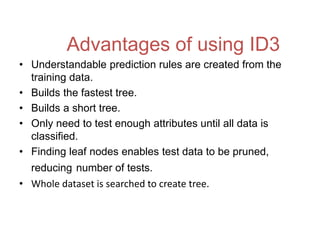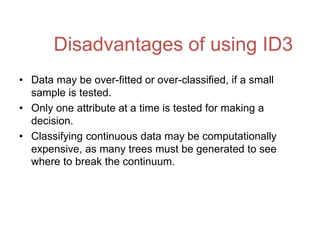1 sur 29

### ID3 ALGORITHM

• 2. ID3 (Iterative Dichotomiser 3): Basic Idea • Invented by J.Ross Quinlan in 1975. • Used to generate a decision tree from a given data set by employing a top-down, greedy search, to test each attribute at every node of the tree. • The resulting tree is used to classify future samples.
• 4. GIVEN TRAINING SET We use non-category attributes to predict the values of category attributes.
• 5. ALGORITHM • Calculate the entropy of every attribute using the data set • Split the set into subsets using the attribute for which entropy is minimum (or, equivalently, information gain is maximum) • Make a decision tree node containing that attribute • Recurse on subsets using remaining attributes
• 6. Entropy • In order to define information gain precisely, we need to discuss entropy first. • A formula to calculate the homogeneity of a sample. • A completely homogeneous sample has entropy of 0 (leaf node). • An equally divided sample has entropy of 1. • The formula for entropy is: • where p(I) is the proportion of S belonging to class I. ∑ is over total outcomes. Log2 is log base 2. Entropy(S) = -p(I) log2 p(I)
• 7. Example 1 • If S is a collection of 14 examples with 9 YES and 5 NO examples then • Entropy(S) = - (9/14) Log2 (9/14) - (5/14) Log2 (5/14) = 0.940
• 8. Information Gain (IG) • The information gain is based on the decrease in entropy after a dataset is split on an attribute. • The formula for calculating information gain is: Gain(S, A) = Entropy(S) - ((|Sv| / |S|) * Entropy(Sv))
• 9. Where: • Sv = subset of S for which attribute A has value v • |Sv| = number of elements in Sv • |S| = number of elements in S
• 10. PROCEDURE • First the entropy of the total dataset is calculated. • The dataset is then split on the different attributes. • The entropy for each branch is calculated. Then it is added proportionally, to get total entropy for the split. • The resulting entropy is subtracted from the entropy before the split. • The result is the Information Gain, or decrease in entropy. • The attribute that yields the largest IG is chosen for the decision node.
• 28. Advantages of using ID3 • Understandable prediction rules are created from the training data. • Builds the fastest tree. • Builds a short tree. • Only need to test enough attributes until all data is classified. • Finding leaf nodes enables test data to be pruned, reducing number of tests. • Whole dataset is searched to create tree.
• 29. Disadvantages of using ID3 • Data may be over-fitted or over-classified, if a small sample is tested. • Only one attribute at a time is tested for making a decision. • Classifying continuous data may be computationally expensive, as many trees must be generated to see where to break the continuum.